# Convert Inches to Feet and Inches in Excel & Google Sheets

This tutorial will demonstrate how to convert values given in inches to feet and inches in Excel & Google Sheets.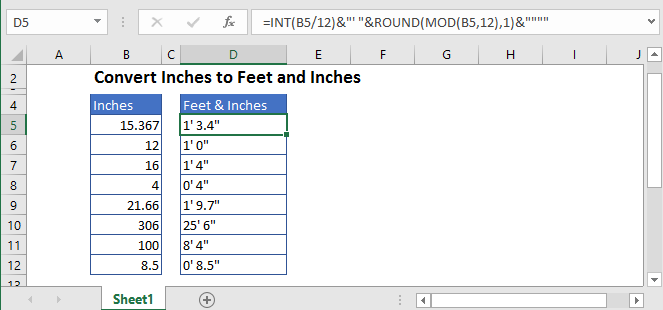## Convert Inches to Feet and Inches

We know that one foot is equal to 12 inches. Thus, if we divide the value given in inches by 12, we’ll the resultant value in feet. But, we want to display the resultant value in feet and inches, together.

So, to show the measurement in feet and inches, in the text form, we’ll use INT and MOD functions and concatenate them.

The formula will be like this:

``=INT(B5/12)&"' "&MOD(B5,12)&""""``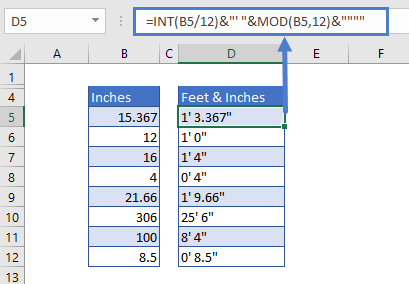### Feet Calculation

In the first part of the formula, the INT function is used to simply return the integer part of the result and leaving any decimal remainder. The integer part gives the resultant value in feet and discards the numbers after the decimal.

``=INT(B5/12)&"’"``

### Inches Calculation

In the second part, the MOD function gives the remainder after division. This gives us the remaining value in inches.

After this, the result is concatenated to a couple of pairs of double-quotes. The outer set of double quotes is required to indicate text, and to show a single double quote in Excel, a set of extra double quotes is required.

``=MOD(B5,12)&""""``

Then both the INT and MOD parts are concatenated together to get the final result in a text value.

## Rounding Inches

We can use a ROUND function before the MOD function to round inches to one decimal value, like this:

``=INT(B5/12)&"' "&ROUND(MOD(B5,12),1)&""""``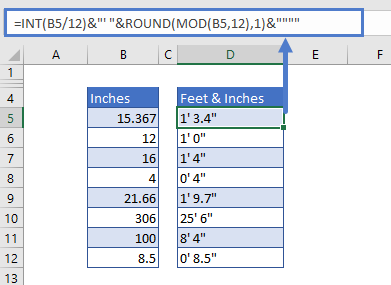## With Labels

We can also put texts between the double quotes after the INT and MOD functions. To display the result in the format of “8 ft. and 4 inches”, we’ll make the following adjustments to the formula:

``=INT(B5/12)&"ft.  "&ROUND(MOD(B5,12),1)&"in."``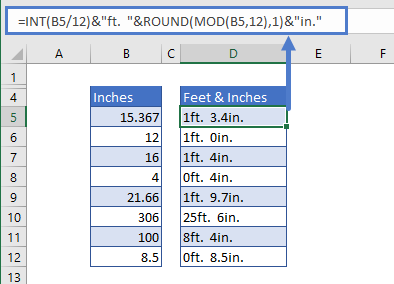## Convert Values in Inches to Feet and Inches in Google Sheets

The conversion of inches to feet and inches using the INT and MOD Functions works the same in Google Sheets as in Excel: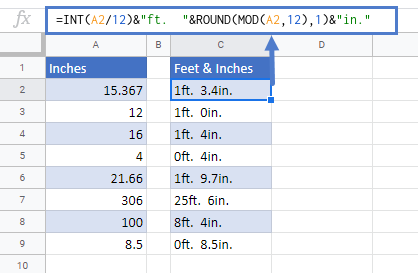### Excel Practice Worksheet

Practice Excel functions and formulas with our 100% free practice worksheets!